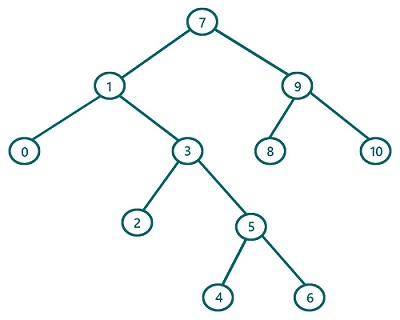# Inorder Traversal in Binary Tree (with recursion) in C, C++

In this article, we are going to find what inorder traversal of a Binary Tree is and how to implement inorder traversal using recursion? We have provided the implementation both in C & C++.
Submitted by Radib Kar, on July 24, 2020

If we classify binary tree traversals, inorder traversal is one of traversal which is based on depth-first search traversal.  The basic concept for inorder traversal lies behind its name. "In" means between and that's why the root is traversed in between its left & right subtree. The basic rule is:

1. First, traverse the left subtree
2. Then traverse the root
3. Finally, traverse the right subtree

Of course, while traversing the subtrees we will follow the same order

So let's traverse the below tree using inorder traversal.For the above tree, the root is: 7

Traverse the left subtree (subtree rooted by 1)

Now to traverse the subtree rooted by 1, it again follows the same set of rules

So, traverse the left subtree of this (rooted by 0)

Since it's a leaf node we can print it as there is no more left subtree. Also, 0 has no right subtree, so this subtree rooted by 0 is traversed totally.

Traversal till now: 0

Since the left subtree of the subtree rooted 1 is traversed, now print the root 1 and traverse the right subtree.

Traversal till now: 0 1

After processing right subtree traversal of the subtree rooted by 1, we will have traversal: 0 1 2 3 4 5 6

Now we have finished the left subtree traversal of the actual tree (rooted by 7)

Now traverse/print 7 and then finally traverse the right subtree in the same way recursively. So ultimately after the whole traversal, we will have: 0 1 2 3 4 5 6 7 8 9 10

The pseudocode would be:

```void inorder(TreeNode root){
if(root is NULL)
return
//recursively traverse left subtree first
inorder(left subtree of root)
//traverse current node
print(root)
// recursively traverse right subtree lastly
inorder(right subtree of root)
}
```

C Implementation:

Tree structure:

```struct tree{
int val;
struct tree* left;
struct tree* right;
};
typedef struct tree TreeNode;
```
```#include <stdio.h>
#include <stdlib.h>

struct tree {

int val;
struct tree* left;
struct tree* right;
};

typedef struct tree TreeNode;

TreeNode* newTree(int data)
{
// Allocate memory for new node
TreeNode* root = (TreeNode*)malloc(sizeof(TreeNode));

// Assign data to this node val
root->val = data;

// Initialize left and right children as NULL
root->left = NULL;
root->right = NULL;
return (root);
}

void inorder(TreeNode* root)
{
//base case
if (root == NULL)
return;
//traverse left sub tree first
inorder(root->left);
//traverse current node secondly
printf("%d ", root->val);
//traverse right sub tree finally
inorder(root->right);
}

int main()
{
//building the tree
TreeNode* t = newTree(7);
t->left = newTree(1);
t->left->left = newTree(0);
t->left->right = newTree(3);
t->left->right->left = newTree(2);
t->left->right->right = newTree(5);
t->left->right->right->left = newTree(4);
t->left->right->right->right = newTree(6);
t->right = newTree(9);
t->right->left = newTree(8);
t->right->right = newTree(10);

printf("Inorder traversal of the above tree is:\n");
inorder(t);

return 0;
}
```

Output:

```Inorder traversal of the above tree is:
0 1 2 3 4 5 6 7 8 9 10
```

C++ implementation:

```#include <bits/stdc++.h>
using namespace std;

class TreeNode { // tree node is defined
public:
int val;
TreeNode* left;
TreeNode* right;
TreeNode(int data)
{
val = data;
left = NULL;
right = NULL;
}
};

void inorder(TreeNode* root)
{
//base case
if (root == NULL)
return;
//traverse left sub tree
inorder(root->left);
//traverse current node secondly
printf("%d ", root->val);
//traverse right sub tree finally
inorder(root->right);
}

int main()
{
//building the tree
TreeNode* t = new TreeNode(7);
t->left = new TreeNode(1);
t->left->left = new TreeNode(0);
t->left->right = new TreeNode(3);
t->left->right->left = new TreeNode(2);
t->left->right->right = new TreeNode(5);
t->left->right->right->left = new TreeNode(4);
t->left->right->right->right = new TreeNode(6);
t->right = new TreeNode(9);
t->right->left = new TreeNode(8);
t->right->right = new TreeNode(10);

printf("Inorder traversal of the above tree is:\n");
inorder(t);

return 0;
}
```

Output:

```Inorder traversal of the above tree is:
0 1 2 3 4 5 6 7 8 9 10
```

What's New

Top Interview Coding Problems/Challenges!

Languages: » C » C++ » C++ STL » Java » Data Structure » C#.Net » Android » Kotlin » SQL
Web Technologies: » PHP » Python » JavaScript » CSS » Ajax » Node.js » Web programming/HTML
Solved programs: » C » C++ » DS » Java » C#
Aptitude que. & ans.: » C » C++ » Java » DBMS
Interview que. & ans.: » C » Embedded C » Java » SEO » HR
CS Subjects: » CS Basics » O.S. » Networks » DBMS » Embedded Systems » Cloud Computing
» Machine learning » CS Organizations » Linux » DOS
More: » Articles » Puzzles » News/Updates